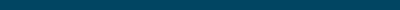# Standard International (SI) Unit Conversion Tool

Use ODX's online unit conversion tool to easily convert between the Standard International units used on your patient's blood test results. Also known as Concentration Molar Conversion.

## Conversion Calculators Library### Clinical Biomarker Unit Conversion Tool

Easily convert between Standard International (SI) and Conventional units on your patient's blood lab test results.### Standard International (SI) Unit Converter

Easily convert between the Standard International units used on your patient's blood test results.### Conventional Unit Converter

Easily convert between the Conventional units used on your patient's blood test results.### eAG/A1C Conversion

Estimated Average Glucose (eAG/A1C) calculator.### WBC Absolute Count

Convert between absolute and % values for Total WBCs, Neutrophils, Lymphocytes, Monocytes, Eosinophils, and Basophils.### Free T3 to Reverse T3 Ratio Calculator

Easily calculate the Free T3 to Reverse T3 Ratio using Free T3 and Reverse T3 values.### Free T3 to Free T4 Ratio Calculator

Easily calculate the Free T3 to Free T4 Ratio using Free T3 and Free T4 values.### LDL to HDL Ratio Calculator

Easily calculate the LDL to HDL Ratio using LDL and HDL values.### AST to ALT Ratio Calculator

Easily calculate the AST to ALT Ratio using AST and ALT values.### Free Testosterone Calculator

Easily calculate the Free Testosterone using albumin, SHBG, and total testosterone values.### Triglyceride-glucose Index Calculator

Easily calculate the Triglyceride-glucose index using triglyceride and fasting-glucose values.